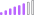cancel
Showing results for
Did you mean:Post Patron

## Count Total Number of Assets Purchased but not Sold

Hi,

I have a table in my data model like this:

 Asset Reference Transaction Type Transaction Date AAA010 Purchase 15-Dec-16 AAA009 Purchase 15-Dec-16 AAA009 Sale 22-Feb-22 AAA008 Purchase 15-Dec-16 AAA007 Purchase 15-Dec-16 AAA006 Purchase 15-Dec-16 AAA006 Sale 28-Apr-22 AAA005 Purchase 15-Dec-16 AAA003 Purchase 15-Dec-16 AAA002 Purchase 15-Dec-16 AAA002 Sale 30-Jul-21 AAA001 Purchase 15-Dec-16

I would like to get a grand total in a card visual of assets that have been purchased but not sold, as per [as of date] which is a date picker the user selects in the report.

So, for example, if the [as of date] = 31/12/2022, from the above there should be a total of 6 assets currently purchased but not sold.

I'm thinking this might need the sum of a filtered count that searches for 'Purchase' or 'Sale' for each asset. But there might be a more efficient way of doing this. Any help appreciated.

1 ACCEPTED SOLUTIONSuper User

Yes, that should work. Try

``````Num unsold items =
VAR ReferenceDate =
MAX ( 'Date'[Date] )
VAR SummaryTable =
VALUES ( 'Table'[Asset Reference] ),
"@purchased",
CALCULATE (
COUNTROWS ( 'Table' ),
'Table'[Transaction Date] <= ReferenceDate,
'Table'[Transaction type] = "Purchase"
),
"@sold",
CALCULATE (
COUNTROWS ( 'Table' ),
'Table'[Transaction Date] <= ReferenceDate,
'Table'[Transaction type] = "Sale"
)
)
RETURN
COUNTROWS ( FILTER ( SummaryTable, [@purchased] > [@sold] ) )
``````
4 REPLIES 4Super User

You could try

``````Num unsold items =
VAR ReferenceDate =
MAX ( 'Date'[Date] )
VAR PurchasedItems =
CALCULATETABLE (
VALUES ( 'Table'[Asset Reference] ),
'Table'[Transaction date] <= ReferenceDate,
'Table'[Transaction type] = "Purchase"
)
VAR SoldItems =
CALCULATETABLE (
VALUES ( 'Table'[Asset Reference] ),
'Table'[Transaction date] <= ReferenceDate,
'Table'[Transaction type] = "Sale"
)
VAR UnsoldItems =
EXCEPT ( PurchasedItems, SoldItems )
RETURN
COUNTROWS ( UnsoldItems )
``````

This should work if an asset can only be bought and sold once. If an asset can be purchased then sold and then repurchased then different code would be needed.Post Patron

Thank you @johnt75

You are right - your solution does work for 99% of assets, but there is the possibilty that an asset could be purchased, sold and then purchased again, and I think there is one case where this is so. Even if not, there could potentially be a case of this in the future.
So I was thinking of some form of count for each asset of 'purchase' transactions, and a count of 'sale' transactions and if purchase is greater than sale then it can be counted as +1.
I am not sure the most efficient way to express it in the DAX though or if that is the best logical way of achieving the goal?Super User

Yes, that should work. Try

``````Num unsold items =
VAR ReferenceDate =
MAX ( 'Date'[Date] )
VAR SummaryTable =
VALUES ( 'Table'[Asset Reference] ),
"@purchased",
CALCULATE (
COUNTROWS ( 'Table' ),
'Table'[Transaction Date] <= ReferenceDate,
'Table'[Transaction type] = "Purchase"
),
"@sold",
CALCULATE (
COUNTROWS ( 'Table' ),
'Table'[Transaction Date] <= ReferenceDate,
'Table'[Transaction type] = "Sale"
)
)
RETURN
COUNTROWS ( FILTER ( SummaryTable, [@purchased] > [@sold] ) )
``````Post Patron

It works perfectly. Thank you for your help!